# RHS

RHS

In triangles, you must have studied about congruency of triangles.

Congruency of triangles means that:

• All corresponding angles are equal.
• All corresponding sides are equal.

However, in order to be sure that the two triangles are congruent, we do not necessarily need to have information about all sides and all angles. We use certain rules to prove the congruency of triangles.

Look at the triangles given below. Can we place these triangles on each other without any gaps or overlaps?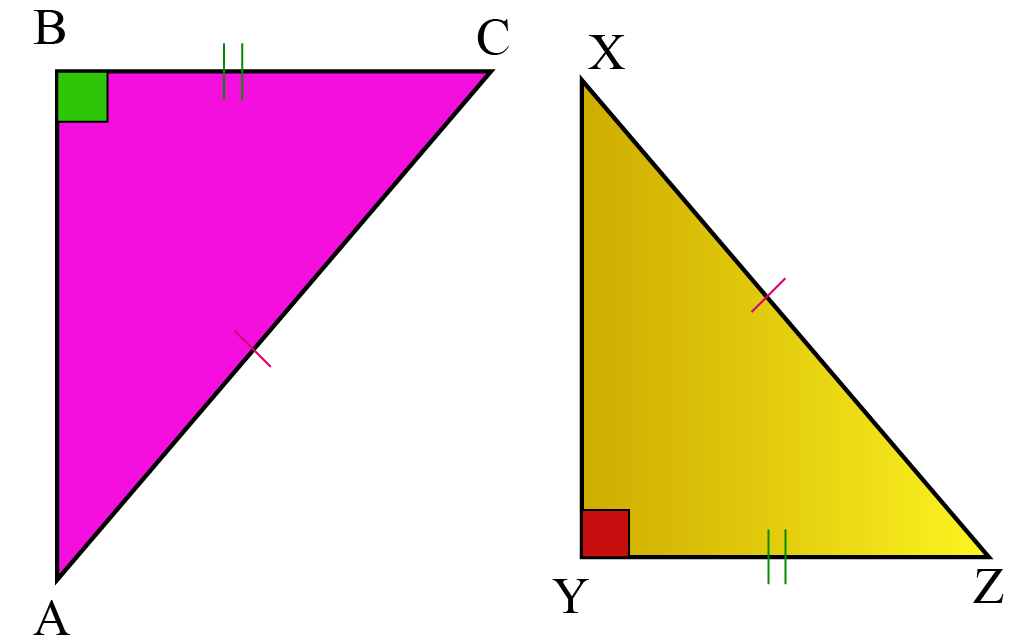In this mini-lesson, we will explore about RHS congruency criterion by learning about its definition and proof with the help some solved examples and a few interactive questions for you to test your understanding.

Let's begin!

## Lesson Plan

 1 What is RHS Congruence Rule in Triangles? 2 Thinking Out of the Box! 3 Important Notes on RHS 4 Solved Examples on RHS 5 Interactive Questions on RHS

## What Is RHS Congruence Rule in Triangles?

RHS criterion of congruence stands for Right Angle-Hypotenuse-Side (full form of RHS congruence).

RHS congruence theorem states that, if the hypotenuse and side of one right-angled triangle are equal to the hypotenuse and the corresponding side of another right-angled triangle, the two triangles are congruent.

This rule is only applicable in right-angled triangles.

An important point to note here is that when we keep hypotenuse and any one of the other 2 sides of two right triangles equal, we are automatically getting three similar sides, as all three sides in a right triangle are related to each other and that relation is popularly known as Pythagoras theorem.

\begin{align} \text{hypotenuse}^2=\text{base}^2+\text{perpendicular}^2 \end{align}

So, in two right triangles, if the length of base and hypotenuse are 3 units and 5 units respectively, then perpendicular of both the triangles is of length 4 units.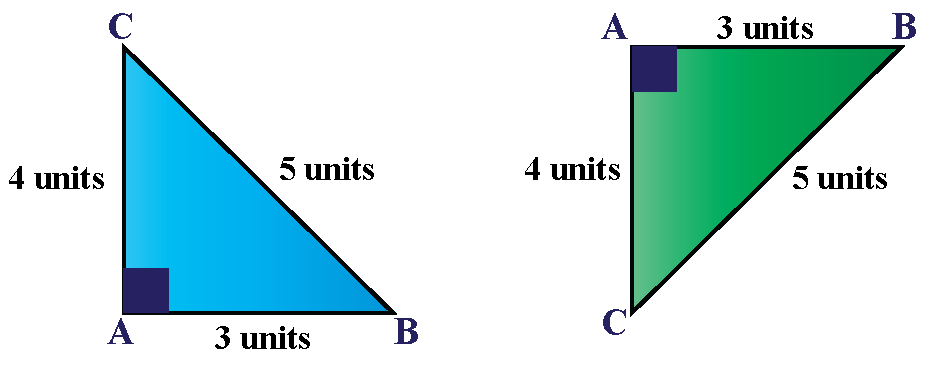Let's see how.

\begin{align} \text{hypotenuse}^2=\text{base}^2+\text{perpendicular}^2 \end{align}

\begin{align} 5^2=3^2+\text{perpendicular}^2 \end{align}

\begin{align} 25=9+\text{perpendicular}^2 \end{align}

\begin{align} 25-9=\text{perpendicular}^2 \end{align}

\begin{align} \text{perpendicular}^2=16 \end{align}

\begin{align} \text{perpendicular}=4\ \text{units} \end{align}Think Tank
1. Under the RHS congruence criterion, we consider two sides and an angle in two right triangles. Under SAS criterion also, we consider two sides and an angle. So, think and tell why do we need to have RHS congruency rule as a separate criterion to prove congruency of triangles?

## What Is the Proof of RHS Congruence Theorem?

Let us do an activity to understand the proof of RHS congruence theorem.

### Proof

Try to draw two triangles $$\triangle ABC$$ and $$\triangle PQR$$ with any one of the angles as $$90^o$$.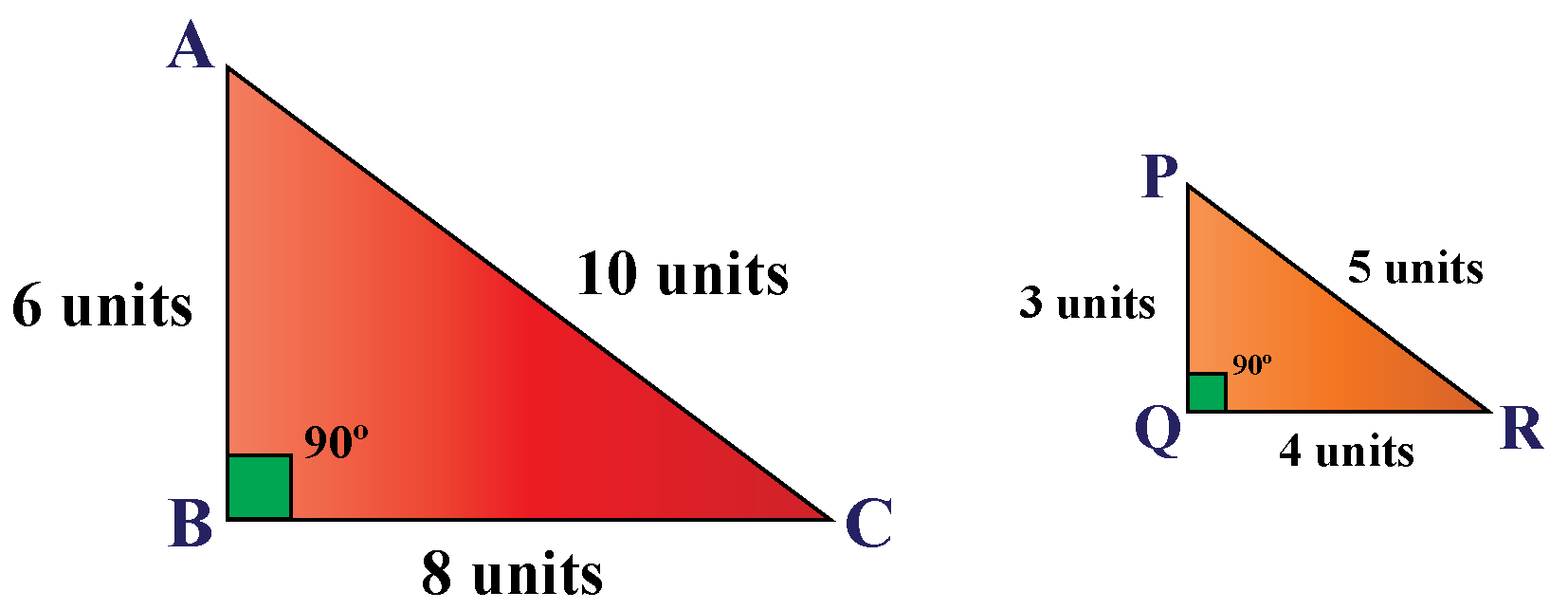Are these triangles congruent?

No.

Now, let's try to keep hypotenuse side equal in both the triangles along with one $$90^o$$ angle.

Let's try to make the hypotenuse side of $$\triangle PQR$$ equals to 10 units.

If we change the hypotenuse of a triangle, other side-lengths will also be changed to maintain the Pythagoras relation between the sides, i.e. $$\text{hypotenuse}^2=\text{base}^2+\text{perpendicular}^2$$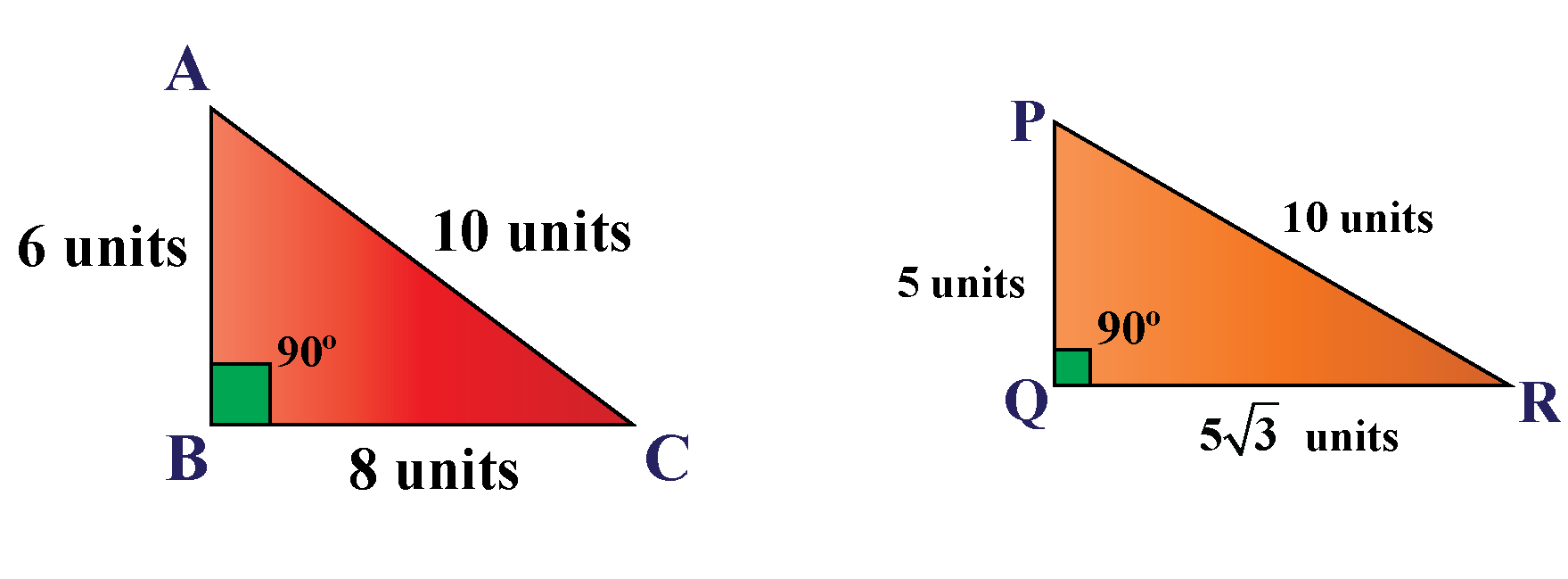Can you see congruent triangles now?

Still no?

Now, let's keep one more side equal in both the triangles and observe the result.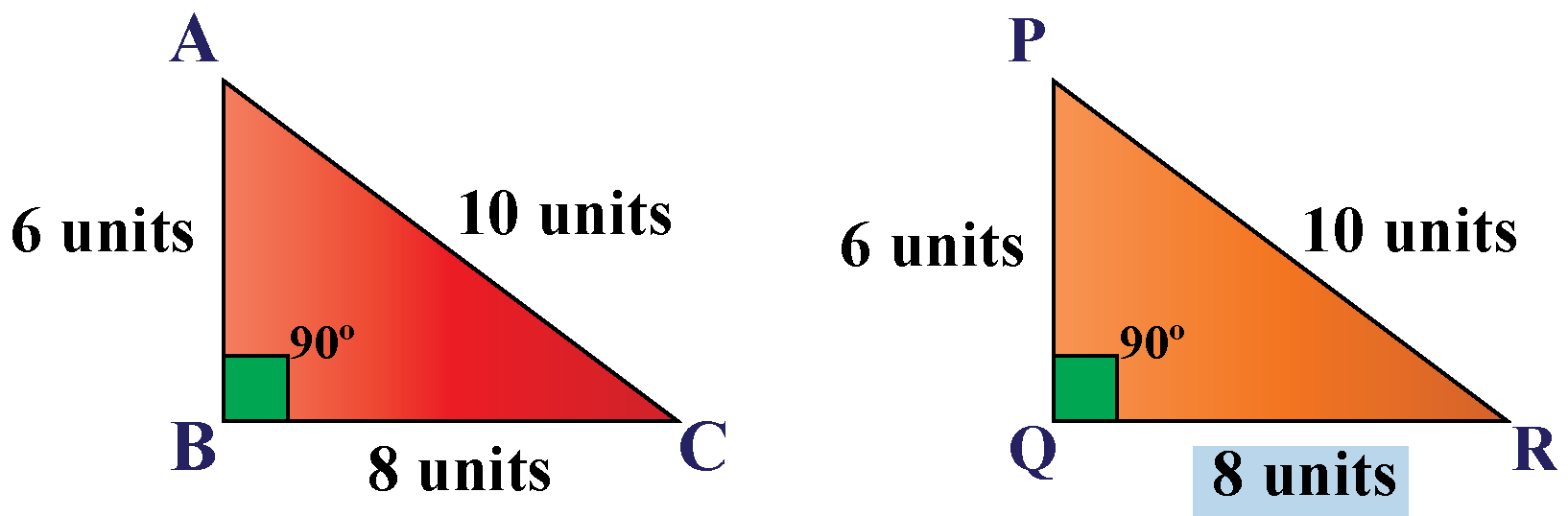Are we getting congruent triangles now?

Yes, in the above image, $$\triangle ABC \cong \triangle PQR$$.

We can place both the triangles on each other without any gaps and overlaps.

Hence, $$\triangle ABC \cong \triangle PQR$$ using RHS congruency rule.

### Example and Solution

State and proof whether the given triangles are congruent or not.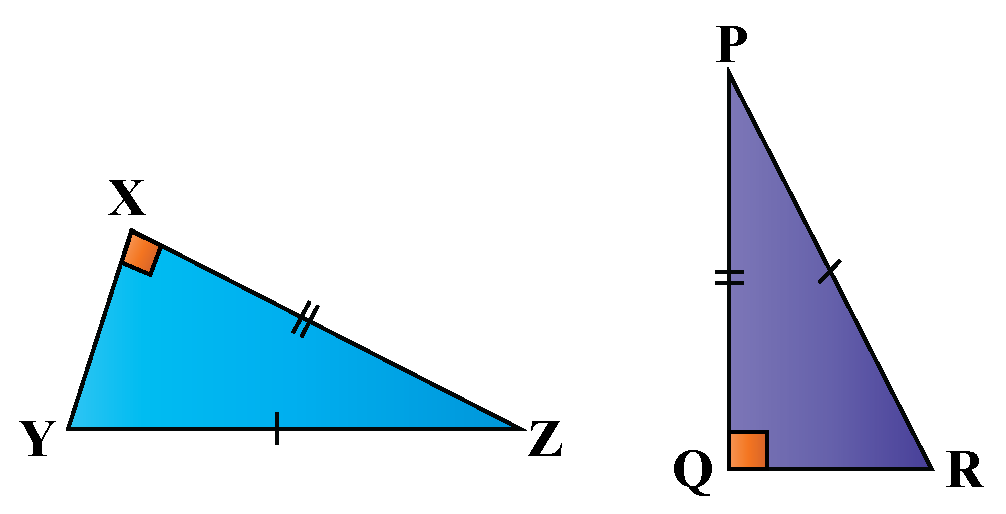In the given triangles, $$\triangle ZXY$$ and $$\triangle PQR$$

$\text{side}\ XZ=\text{side}\ PQ$

$\text{side}\ YZ=\text{side}\ PR$

$\angle ZXY=\angle PQR=90^o$

$$\therefore \triangle ZXY \cong \triangle PQR$$, by RHS congruence criterion.